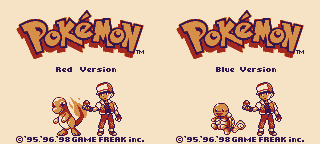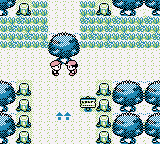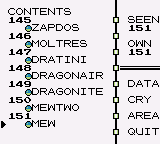# Tutorial #3 - Bit Flags

## Overview

This tutorial will show a few examples of how to create achievements for a game which has a lot of Bit Flags. Pokémon Red|Blue was chosen as the tutorial example because all the defeated Trainers, Item pickups, and caught Pokémon are tracked with Bit Flags.## For Loops

The following examples use variations of For Loops to programmatically cycle through an Array of Bit Flags. For Loops are an extremely useful programming concept that allow you to execute a block of code any number of times. Using loops you can create very complex achievements in a few lines of code. If you are unfamiliar with the concept of For Loops then please review the Khan Academy video For Loops! A New Kind of Loop

## Arrays

Arrays are sequential data, of a single data type, that can be accessed with a numerical index. In RAScripts Arrays can be numbers, text, conditions, or another nested Array. Arrays work well together with For Loops since you can loop through an Array generating new code for each element in the Array. If you are unfamiliar with the concept of Arrays then please review the Khan Academy video Intro to Arrays

## Example 3A: Setting the Forest Ablaze

Pokémon Red|Blue is a Role Playing Game (RPG) where you collect and train Pokémon to fight other Pokémon Trainers around the Kanto region. You also collect or buy special Items that can heal your Pokémon, remedy status affects, or help your Pokémon evolve. Both Trainer fights and Item pick ups are tracked in-game using Bit Flags. The following achievement is for when the player fights all of the Trainers and picks up all of the Items in the Viridian Forest. The Viridian Forest area was selected since it was one of the smaller sections of the game however, you can use the same snippet of code for any section of the game by passing a different Array of Bit Flags. It doesn’t matter if there are more or less Bit Flags than the example below since the code will adapt to the number of elements in the Array by using the `length()` function.``````// Pokémon Red Version | Pokémon Blue Version
// #ID = 724

// An array on all the bitflags in the Viridian Forest
ViridianForest = [
bit0(0xd6f0), // Hidden Potion
bit1(0xd6f0), // Hidden Antidote
bit4(0xd5b2), // Antidote
bit5(0xd5b2), // Potion
bit6(0xd5b2), // Poke Ball
bit2(0xd7f3), // Bug Catcher 01
bit3(0xd7f3), // Bug Catcher 02
bit4(0xd7f3)  // Bug Catcher 03
]

// Check if array of passed flags goes from previously all but one flag is true
// to all flags being true.
function FlagsComplete(Flags)
{
return sum_of(Flags, f => prev(f)) == length(Flags) - 1 &&
measured(sum_of(Flags, f => f) == length(Flags))
}

// Make an achievement for finding every item and defeating every trainer
// in the Viridian Forest
achievement(
title = "Example 3A: Setting the Forest Ablaze",
description = "Find every Item and defeat every Trainer in the Viridian Forest.",
points = 0,
trigger = FlagsComplete(ViridianForest)
)
``````

### sum_of

The above code utilizes the command sum_of to add the value of each Bit Flag in the Array. Since a bit is 1 if on and 0 if off the summation of the Bit Flags will tell you how many Bit Flags are on. Using the length of 8 for the Array in this example we test if the previous number of Bit Flags was 7 and the current number of bit flags that are on is 8. The advantage of counting Bit Flags like this is that the player can collect the Items and fight the Trainers in any order and still get the achievement. Additionally, we get save protection since the player must increment the Bit Flag count to get the achievement and it won’t trigger if they load a save file with the Bit Flags all on. To help understand how to use sum_of it might help to deconstruct an example. Consider the line:

``````sum_of(Flags, f => prev(f))
``````

The above line could be written as a For Loop where `Flags` is the array we are looping through and `f` is each element in the array. The following code is equivalent to the above:

``````sum = 0
for f in Flags
{
sum = sum + prev(f)
}
// The value of sum if the number of bit flags that were on in the previous frame.
``````

Note that the `f => prev(f)` part of the sum_of means that the previous value of each element is counted. If we wrote `f => f` then the current value of each element is counted.

### Unrolling a Loop

To better understand how the For Loop is interpreted by the compiler you can unroll the loop into individual lines of code. Unrolling a loop is a technique used by programmers to optimize code since it avoids conditional jumps which take a small amount resources. The technique is more useful on embedded hardware where resources are extremely limited however, it is a useful exercise when learning For Loops. Consider the same loop from above:

``````sum = 0
for f in Flags
{
sum = sum + prev(f)
}
``````

To unroll this For Loop we would copy the line of code inside of the eight times, one for each element in the Array and replace f with the element.

``````sum = 0
sum = sum + prev(bit0(0xd6f0)) // Hidden Potion
sum = sum + prev(bit1(0xd6f0)) // Hidden Antidote
sum = sum + prev(bit4(0xd5b2)) // Antidote
sum = sum + prev(bit5(0xd5b2)) // Potion
sum = sum + prev(bit6(0xd5b2)) // Poke Ball
sum = sum + prev(bit2(0xd7f3)) // Bug Catcher 01
sum = sum + prev(bit3(0xd7f3)) // Bug Catcher 02
sum = sum + prev(bit4(0xd7f3)) // Bug Catcher 03
``````

Both code snippets are equivalent however, the one using the For Loop is easier to write and there is less chance of a typo in the code. For Loops become even more desirable when the number of elements in the Array increases.

Scripts: Example #3A script

## Example 3B: Gotta ACTUALLY Catch ‘em All

The main gimmick of Pokémon is that you can catch and train any of the 151 Pokémon in the game. This is emphasized by the games’ catch phrase “Gotta Catch ‘em All”. The game tracks both which Pokémon you have seen and/or caught in the Pokédex. When it was released Pokémon was sold as a Red and Blue version, each version with its own game exclusive Pokémon. So to actually catch all of the Pokémon you needed to trade Pokémon with someone else who had a different version of the game. Additionally some Pokémon like Mew require glitches to catch them. Because of the requirement to trade with another person or use glitches the achievement this example is based on is from the bonus set. The reason why it was chosen as one of the examples is because catching all 151 Pokémon is tracked in consecutive Bit Flags which allows for some interesting optimizations.``````// Pokémon Red Version | Pokémon Blue Version
// #ID = 724

// \$C06E: Music Playng, title screen = 4105
function TitleMusic() => word(0x00C06E) == 4105

// Count each bit between \$D2F7 bit 0 to \$D309 bit 6
// these are the consecutive bitflags for each of the
// 151 Pokémon in the Pokédex
function PokedexCount()
{
count = 0
for address in range(0xD2F7, 0xD308) {
}
count = count + sum_of(range(0,6), b => bit(b,0xD309))
return count
}

// Create an achievement for when the player goes from
// 150 Pokémon to 151 Pokémon in the Pokédex
achievement(
title = "Example 3B: Gotta ACTUALLY Catch 'em All",
description = "Catch all 151 Pokémon. Yes ALL of them.",
points = 0,
trigger = never(TitleMusic()) &&
prev(PokedexCount()) == 150 &&
measured(PokedexCount() == 151)
)
``````

### bitcount

The above example uses the fact that the Pokédex uses consecutive bits to optimize the code with bitcount. Instead of adding the bits together with a For Loop, bitcount counts the bits with one command. Consider line:

``````count = count + bitcount(address)
``````

The bits could also be counted using the sum_of function like:

``````count = count + sum_of(range(0,7), b => bit(b, address))
``````

Note that by using bitcount instead of sum_of we drastically reduce that number of lines generated by the script. Instead of adding each bit per line we add eight bits per line. This results in 51 lines using bitcount instead of 303 lines using sum_of, approximately 6x smaller.

### sum_of bits

Another interesting trick in this example is the use of sum_of to add the last seven bits of the Pokédex. Since we want to ignore the value of the eighth bit we can use sum_of to add the values of bits zero to six. Note that the command `range(0,6)` is a shortcut function which returns an Array of numbers, in this case `[0, 1, 2, 3, 4, 5, 6]` . Consider the line:

``````count = count + sum_of(range(0,6), b => bit(b,0xD309))
``````

To help understand what going on we will unroll it like the previous example.

``````count = count + bit(0,0xD309)
count = count + bit(1,0xD309)
count = count + bit(2,0xD309)
count = count + bit(3,0xD309)
count = count + bit(4,0xD309)
count = count + bit(5,0xD309)
count = count + bit(6,0xD309)
``````

By using For Loops we can make very complex achievements quickly and efficiently.

Scripts: Example #3B script

## Example 3C: Assemble the Dream Team

When battling other Trainers you can choose up to six Pokémon to fight for you. One of the most enjoyable aspects of Pokémon is the ability to mix and match your team. By learning the strengths and weakness of each Pokémon type you can assemble your team to counter an opponent’s Team.## Homework #3

A) Pick your six favorite Pokémon available in Pokémon Red | Blue. B) Using the Pokedex memory of Bit Flags between \$D2F7 bit 0 to \$D309 bit 6 create an achievement for catching all six Pokémon selected in part A

Solutions: Tutorial #3 Solution

All RAScript Tutorials can be found here.